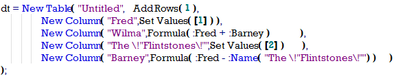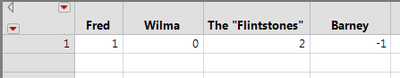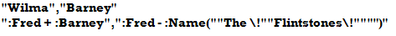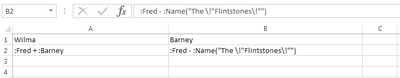World Statistics Day was yesterday, but we’re celebrating all week long! This celebration means acknowledging the impact statistics has on our world. Who is your favorite statistician? Share with us who they are and why they top your favorites list.
Choose Language Hide Translation Bar

## Column Formulas to CSV

JSL to find the formulas in a JMP data table and write a CSV (comma separated value) file.  There is an open issue for exporting formulas to Excel; maybe this script can serve as a starting point to determine what is really needed.

The code also demonstrates a simple use of Regex, user functions, and saving a file to the \$TEMP directory.

This code has only been tested on the included data table.which opens this tablewhich the attached JSL exports to this CSV textIf the .CSV file is opened in Excel, the formulas are character fields.Another option is to add a row to the table where any column formulas could be appended.

The following example loops through each column to check for a formula.  If a formula is found, the formula is added to the last row.  Finally, the table is stored as CSV or Excel.

``````dt = Open( "\$SAMPLE_DATA\Tiretread.jmp" );
dt << Run Formulas;
dt << Add Rows( 1 );
r = N Rows( dt );
For( i = 1, i <= N Cols( dt ), i++,
f = Column( i ) << Get formula;
If( !Is Empty( f ),
Column( i ) << Delete Formula;
Column( i ) << Data Type( "Character" );
Column( i )[r] = Char( Name Expr( f ) );
);
);

Close( dt, "No Save" );``````

Is this helpful for exporting JMP column formulas to Excel?

Much better approach, thanks!  You've used JMP's builtin functions to do a lot of the stuff I did the hard way.

Article Labels

There are no labels assigned to this post.

Article Tags
Contributors